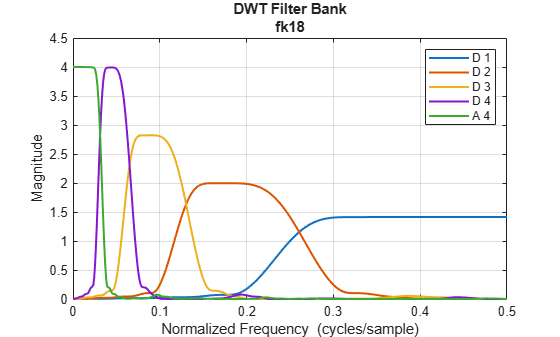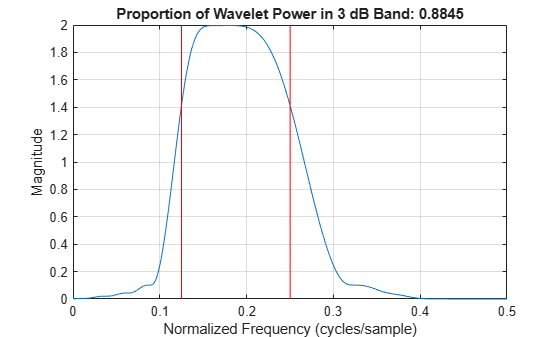Main Content

# powerbw

DWT filter bank power bandwidth

## Syntax

``bwtable = powerbw(fb)``

## Description

example

````bwtable = powerbw(fb)` returns a MATLAB® table `bwtable` containing the theoretical and measured bandwidths of the discrete wavelet transform (DWT) filter bank `fb`. The table contains the following variables by level: DWT frequency bandsMeasured wavelet and scaling filter 3 dB bandwidthsProportions of the total energy in the reported bands ```

## Examples

collapse all

Obtain the 3 dB bandwidths of a level-4 discrete wavelet transform with the Fejér-Korovkin `fk18` wavelet. Obtain the frequency responses of the wavelets. Plot the one-sided frequency responses for the wavelet filters.

```fb = dwtfilterbank('Wavelet','fk18','Level',4); bw = powerbw(fb); [psidft,f] = freqz(fb); freqz(fb)```Choose the wavelet bandpass filter whose peak magnitude is equal to 2. Obtain the lower and upper bounds of the 3 dB bandwidth of the filter.

```wv = 2; wvBw = bw.Wavelet3dBBandwidth(wv,:);```

Plot the magnitude frequency response of the filter and the 3 dB limits. Since the frequency response has a maximum value equal to 2, confirm the lower and upper frequency bounds intersect the frequency response at $\sqrt{2}$.

```filLength = size(psidft,2); plot(f(filLength/2+1:end),abs(psidft(wv,filLength/2+1:end))) hold on plot([wvBw(1) wvBw(1)],[0 2],'r') plot([wvBw(2) wvBw(2)],[0 2],'r') grid on title(['Proportion of Wavelet Power in 3 dB Band: ',num2str(bw.WaveletPowerIn3dBBand(wv))]) xlabel('Normalized Frequency (cycles/sample)') ylabel('Magnitude')```## Input Arguments

collapse all

Discrete wavelet transform (DWT) filter bank, specified as a `dwtfilterbank` object.

## Output Arguments

collapse all

Theoretical and measured bandwidths of the DWT filter bank `fb`, returned as a MATLAB table. `bwtable` is L-by-8, where L is the wavelet transform level of the filter bank. Levels are ordered by decreasing resolution. `bwtable` has the following eight variables:

Level of DWT decomposition, returned as a positive integer.

Theoretical DWT frequency bands by level, returned as a two-element real-valued vector.

Measured wavelet 3 dB bandwidths by level, returned as a two-element real-valued vector.

Measured scaling filter 3 dB bandwidths by level, returned as a two-element real-valued vector.

Proportion of total wavelet power in the measured 3 dB band by level, returned as a positive scalar.

Proportion of total scaling filter power in the measured 3 dB band by level, returned as a positive scalar.

Proportion of total wavelet power in the theoretical DWT band by level, returned as a positive scalar.

Proportion of total scaling filter power in the theoretical DWT band by level, returned as a positive scalar.

## See Also

Introduced in R2018a

## SupportGet trial now